# Basic Arithmetic : Laws of Exponents

## Example Questions

### Example Question #1 : Operations In Expressions

Simplify: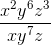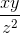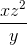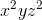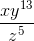Explanation:

When dividing terms with the same bases, remember to subtract the exponents.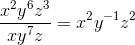Keep in mind that when there is a negative exponent in the numerator, putting that term in the denominator will make the exponent positive.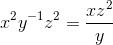### Example Question #1 : Laws Of Exponents

Evaluate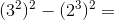Explanation:

We first need to apply the Exponent Rule to our two terms.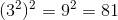and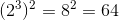.

Then we do subtraction to obtain our final answer,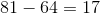.

### Example Question #122 : Basic Arithmetic

Simplify the following expression: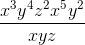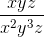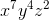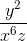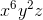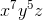Explanation:

The correct answer isdue to the law of exponents. When solving this type of problem, it is easiest to focus on like terms (i.e. terms containing x or terms containing y).

First we can start by simplifying the 'x' terms. We start with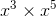which is equivalent to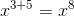. We then are left with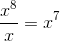.

Now we can simplify the 'y' terms as follows: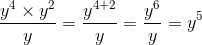.

Last, the 'z' terms can be simplified as follows: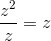.

This leaves us with the final simplified answer of.

### Example Question #2 : Laws Of Exponents

Which of the following is equivalent to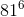?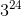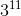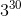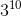Explanation:

Since all of the answer choices look like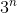, let's findin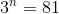.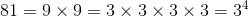Then,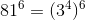When you have an exponent being raised to an exponent, multiply the exponents together.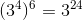### Example Question #1 : Laws Of Exponents

What is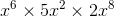?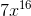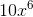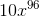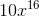Explanation:

When terms with the same base are multiplied, multiply the coefficients together then add up all the exponents.

For the coefficients: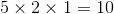For the exponents: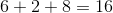Thus, the answer is### Example Question #1 : Laws Of Exponents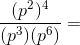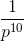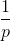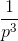Explanation:

Start by simplifying the numerator.

When an exponent is raised to another exponent, multiply the two exponents together.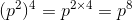Now, tackle the denominator. When two numbers of the same base are multiplied, you want to add the exponents.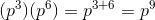Put the numerator and denominator together: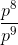When you have a fraction with terms that have the same base, you want to subtract the exponent in the denominator from the exponent in the numerator.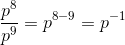To make a term with a negative exponent in the numerator positive, put it in the denominator.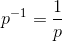### All Basic Arithmetic Resources